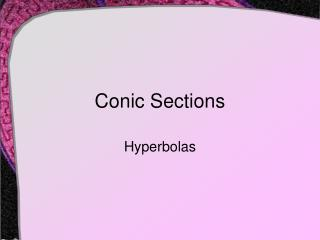DownloadDownload PresentationConic Sections

# Conic Sections

Download Presentation## Conic Sections

- - - - - - - - - - - - - - - - - - - - - - - - - - - E N D - - - - - - - - - - - - - - - - - - - - - - - - - - -
##### Presentation Transcript

1. Conic Sections Hyperbolas

2. Definition • The conic section formed by a plane which intersects both of the right conical surfaces • Formed whenor when the plane isparallel to the axis of the cone

3. Definition • A hyperbola is the set of all points in the plane where • The difference between the distances • From two fixed points (foci) • Is a constant Geogebra Demonstration

4. Experimenting with Definition • Turn on Explore geometric definition. A purple point will appear on the hyperbola, along with two line segments labeled L1 and L2. Drag the purple point around the hyperbola. • Do the lengths of L1 and L2 change? • What do you notice about the absolute value of the differences of the lengths? • How do these observations relate to the geometric definition of a hyperbola. • Observe the values of L1, L2, and the difference | L1 − L2 | as you vary the values of a and b. • How is the difference | L1 − L2 | related to the values of a and/or b? (Hint: Think about multiples.) • Determine the difference | L1 − L2 | for a hyperbola where a = 3 and b = 4. Use the Gizmo to check your answer.

5. Elements of An Ellipse • Transverse axis • Line joining the intercepts • Conjugate axis • Passes throughcenter, perpendicularto transverse axis • Vertices • Points where hyperbola intersects transverse axis

6. Elements of An Ellipse • Transverse Axis • Length = 2a • Foci • Location (-c, 0), (c, 0) • Asymptotes • Experiment with Pythagorean relationship

7. Equations of An Ellipse • Given equations of ellipse • Centered at origin • Opening right and left • Equations of asymptotes • Opening up and down • Equations of asymptotes

8. Try It Out • Find • The center • Vertices • Foci • Asymptotes

9. More Trials and Tribulations • Find the equation in standard form of the hyperbola that satisfies the stated conditions. • Vertices at (0, 2) and (0, -2), foci (0, 3) and (0, -3) • Foci (1, -2) and (7, -2)slope of an asymptote = 5/4

10. Assignment • Hyperbola A • Exercise set 6.3 • Exercises 1 – 25 oddand 33 – 45 odd

11. Conic Sections The Return of the Hyperbola

12. Eccentricity of a Hyperbola • The hyperbola can be wide or narrow

13. Eccentricity of a Hyperbola • As with eccentricity of an hyperbola, the formula is • Note that for hyperbolas c > a • Thus eccentricity > 1

14. Try It Out • If the vertices are (1, 6) and (1, 8) and the eccentricity is 5/2 • Find the equation (standard form) of the hyperbola • The center of the hyperbola is at (-3, -3) and the conjugate axis has length 6, and the eccentricity = 2 • Find two possible hyperbola equations

15. Application – Locating Position • For any point on a hyperbolic curve • Difference between distances to foci is constant. • Result: hyperbolas can be used to locate enemy guns “If the sound of an enemy gun is heard at two listening posts and the difference in time is calculated, then the gun is known to be located on a particular hyperbola.  A third listening post will determine a second hyperbola, and then the gun emplacement can be spotted as the intersection of the two hyperbolas.”

16. Application – Locating Position • The loran system navigator • equipped with a map that gives curves, called loran lines of position. • Navigators find the time interval between these curves, • Narrow down the area that their craft's position is in. • Then switch to a different pair of loran transmitters • Repeat the procedure • Find another curve representing the craft's position.

17. Construction • Consider a the blue string • Keep markeragainst rulerand with stringtight • Keep end ofruler on focusF1 , string tied to other end

18. Graphing a Hyperbola on the TI • As with the ellipse, the hyperbola is not a function • Possible to solve for y • Get two expressions • Graph each • What happensif it opensright and left?

19. Graphing a Hyperbola on the TI • Top and bottom of hyperbola branches are graphed separately • As with ellipsesyou must Ellipse with Geogebra

20. Assignment • Hyperbolas 2 • Exercise Set 6.3 • Exercises 27 – 31 oddand 49 – 63 odd